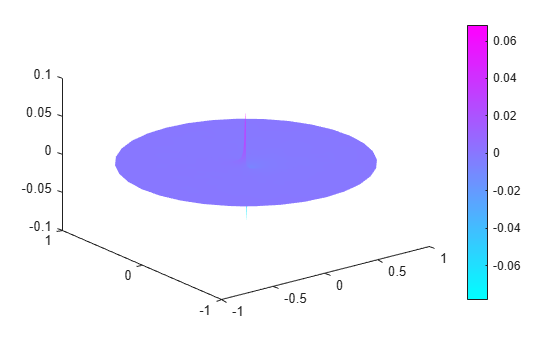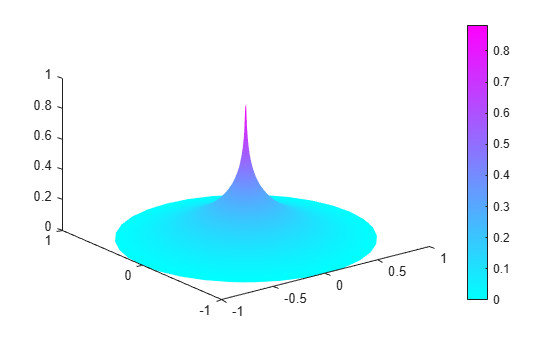# Poisson's Equation with Point Source and Adaptive Mesh Refinement

This example shows how to solve a Poisson's equation with a delta-function point source on the unit disk using the `adaptmesh` function.

Specifically, solve the Poisson's equation

`$-\Delta u=\delta \left(x,y\right)$`

on the unit disk with zero Dirichlet boundary conditions. The exact solution expressed in polar coordinates is

`$u\left(r,\theta \right)=\frac{\mathrm{log}\left(r\right)}{2\pi },$`

which is singular at the origin.

By using adaptive mesh refinement, Partial Equation Toolbox™ can accurately find the solution everywhere away from the origin.

The following variables define the problem:

• `c`, `a`: The coefficients of the PDE.

• `f`: A function that captures a point source at the origin. It returns 1/area for the triangle containing the origin and 0 for other triangles.

```c = 1; a = 0; f = @circlef;```

Create a PDE Model with a single dependent variable.

```numberOfPDE = 1; model = createpde(numberOfPDE); ```

Create a geometry and include it in the model. For more information, see the |circleg| and |pdegeom| pages.

```g = @circleg; geometryFromEdges(model,g);```

Plot the geometry and display the edge labels.

```figure; pdegplot(model,'EdgeLabels','on'); axis equal title 'Geometry With Edge Labels Displayed';```Specify the zero solution at all four outer edges of the circle.

`applyBoundaryCondition(model,'dirichlet','Edge',(1:4),'u',0);`

`adaptmesh` solves elliptic PDEs using the adaptive mesh generation. The 'tripick' parameter lets you specify a function that returns which triangles will be refined in the next iteration. `circlepick` returns triangles with computed error estimates greater a given tolerance. The tolerance is provided to `circlepick` using the 'par' parameter.

`[u,p,e,t] = adaptmesh(g,model,c,a,f,'tripick','circlepick','maxt',2000,'par',1e-3);`
```Number of triangles: 258 Number of triangles: 515 Number of triangles: 747 Number of triangles: 1003 Number of triangles: 1243 Number of triangles: 1481 Number of triangles: 1705 Number of triangles: 1943 Number of triangles: 2155 Maximum number of triangles obtained. ```

Plot the finest mesh.

```figure; pdemesh(p,e,t); axis equal```Plot the error values.

```x = p(1,:)'; y = p(2,:)'; r = sqrt(x.^2+y.^2); uu = -log(r)/2/pi; figure; pdeplot(p,e,t,'XYData',u-uu,'ZData',u-uu,'Mesh','off');```Plot the FEM solution on the finest mesh.

```figure; pdeplot(p,e,t,'XYData',u,'ZData',u,'Mesh','off');```##### SupportGet trial now